# Interfacing NTC Thermistor with Arduino

Published  May 6, 2022   0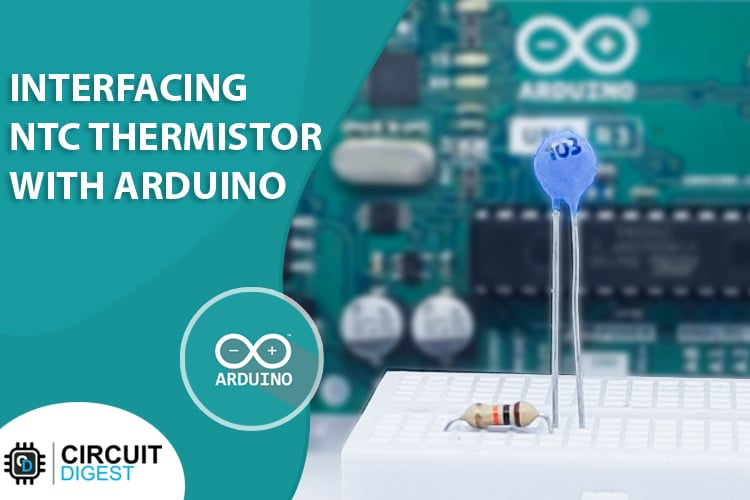Thermistors are simple, inexpensive, and accurate components that make it easy to get temperature data for your projects. Thermistors or THERMally sensitive resISTORs are variable resistors, whose resistance will be changed with the temperature. This feature enables us to read the temperature or change in temperature by measuring the resistance of the thermistors. Thermistors are used in various everyday use items like Thermostats, SMPS, surge protection circuits and rechargeable battery packs, etc.

### NTC Thermistor Pinout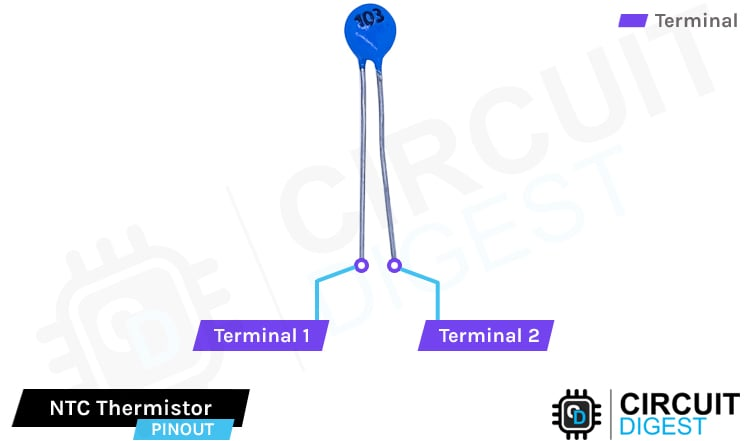Terminal 1 NTC Thermistor terminal 1

Terminal 2 NTC Thermistor terminal 2

### Types of Thermistors

Depending on the materials used and how they react with the temperature thermistors are classified into two types:

• Negative Temperature Coefficient Thermistors or NTC Thermistors
• Positive Temperature Coefficient Thermistors or PTC Thermistors

Positive Temperature Coefficient Thermistors or PTC Thermistors

With PTC thermistors, resistance increases as the temperature rises, usually due to increased Thermal lattice agitations. They are usually used for overcurrent protection, as resettable fuses.

Negative Temperature Coefficient Thermistors or NTC Thermistors

With NTC thermistors, resistance decreases as temperature rises, due to an increase in the number of conduction electrons energized by the thermal agitation from the valance band. NTC are commonly used as temperature sensors or in series with circuits like power supplies as an inrush current limiter.Here is the Resistance Versus Temperature Response graph for the TCS610 10K NTC from wavelength electronics. You can see that at 25⁰C the Resistance of the NTC thermistor is 10Kohms. When the temperature increases the resistance decreases.

### How Does an NTC Thermistor Work?

As already mentioned, the resistance of an NTC thermistor will change with a change in temperature. So, we can measure the resistance of the thermistor and can calculate the temperature in relation to the measured resistance. The thermistor of course is not the best one for accurate temperature measurements, but it is damn cheap and can be used very easily. Here is a small animation showing the working of an NTC thermistor.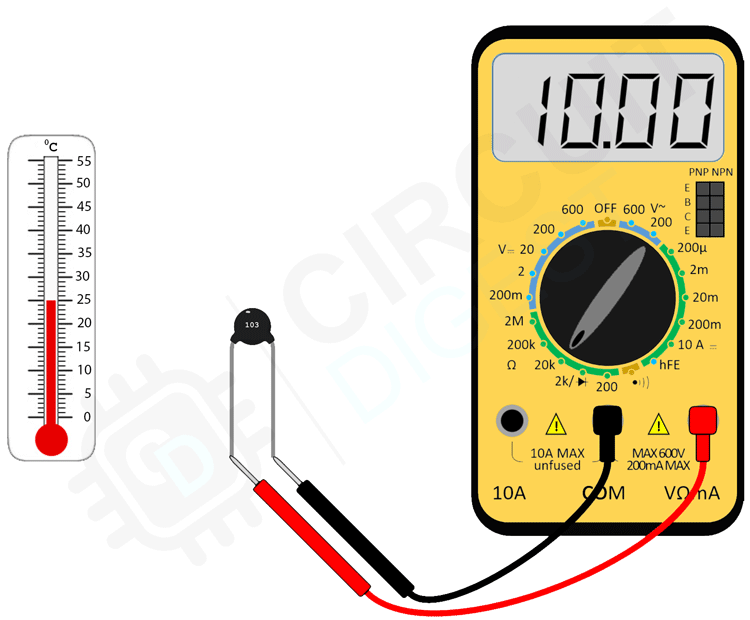What is an NTC Thermistor?

NTC Thermistor is a thermally sensitive resistor with a Negative Temperature Coefficient. Its resistance will decrease when the temperature increases.

How NTC is calculated?

The actual resistance values of a particular NTC thermistor are obtained by multiplying the ratio RT/R25 (tabulated value) by the resistance value at 25 °C, which is specified in the datasheet.

What are NTC Thermistors made of?

Thermistors are made of sintered ceramics consisting of highly sensitive material with consistently reproducible properties of resistance versus temperature.

Are NTC Thermistors Linear?

No. Thermistors are non-linear variable resistance devices.

Why NTC Thermistors are used in Li-ion Battery Packs?

NTC thermistors are used for measuring the battery temperature. Since Li-ion batteries are highly dangerous at high temperatures it is necessary to monitor their temperature continuously.

### Measuring NTC Resistance and Temperature

For measuring the resistance of an NTC thermistor, we will use a voltage divider. One terminal of the thermistor will be connected to the VCC line through a known value resistor and the other terminal will be connected to the ground. The connections will be as shown below.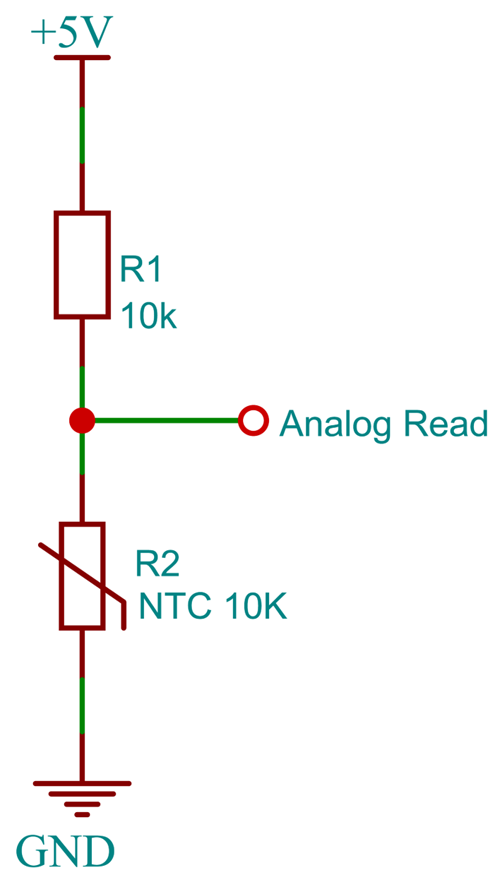To measure the resistance of the NTC Thermistor, first, we will measure the voltage from the voltage divider. And the voltage divider equation is as follows.

`Vout = Vin*[R2/(R1+R2)]`

Since we know Vin, R1, and Vout we can calculate the value of the NTC thermistor R2 with the following equation

`R2=(Vout*R1) / (Vin-Vout)`

### Arduino NTC Thermistor Interfacing Circuit Diagram

The connection is pretty simple. We are going to create a voltage divider with the NTC thermistor and a known value resistor. For this instance, we are going to use a 10K resistor for that. The voltage divider is built with the 10K resistor connected to the 5V and the Thermistor is connected to the ground, The voltage is measured where the NTC thermistor and the resistor are connected together. As we know the value of the reference resistor and the reference voltage, we can calculate the resistance of the thermistor from the measured voltage drop. From this voltage drop, we can then calculate the resistance of the thermistor. From the calculated thermistor resistance, we can also calculate the temperature too.You might be wondering why we have connected the reference resistor to the GPIO2 instead of the 5V pin. It is because of the self _heating phenomena. If we connect a 10K thermistor with a 10K resistor to form a voltage divider, and the voltage divider is connected to the 5V and then ground, we will get a current minimum current flow of about 25mA (5V / (10K + 10K) = 0.25mA) all the time.  Even if this doesn’t seem like a lot of currents, this will, definitely heat up your thermistor because even the 25mA current flow can cause a power dissipation of about 0.625mW (0.25mAx 2.5V). That’s too if we consider if the value of the thermistor and the reference resistor stays constant. As we know the resistance of the thermistor will vary drastically with the temperature. So, allowing continuous current flow will affect the reading. So, in order to avoid it, we will only turn the GPIO to high while we are taking the reading and as soon as we take the reading, we will change the GIO state to low. The actual connection is shown in the below image.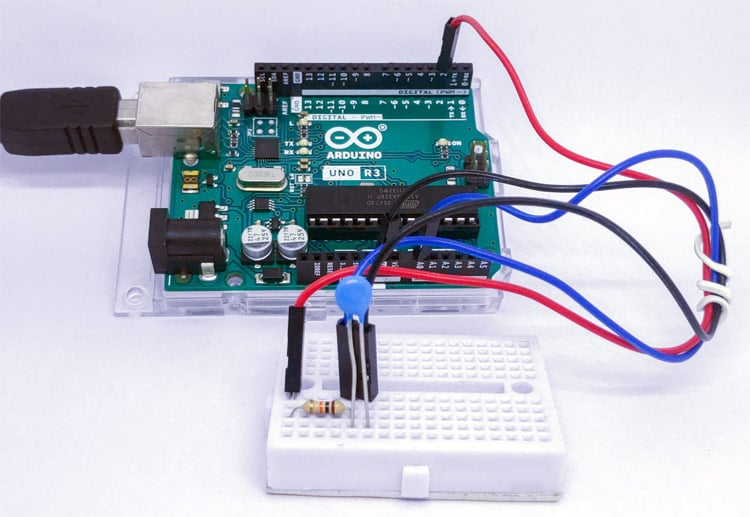### Arduino Code to Interface NTC Thermistor with Arduino

Let’s look at the code section. The code is pretty straightforward. The NTC thermistor resistance is measured using the voltage divider and then the temperature is calculated from it.

In the first lines, we have declared the global variables and the pins. Analog pin A0 is used to measure the voltage at the voltage divider. Pin D2 is used to provide 5V for the voltage divider. The nominal resistance of the NTC at 25⁰C, the Beta coefficient of the NTC, and the resistance of the known value resistor were also declared. Sampling rate is the number of samples taken for the averaging.

```#define ntc_pin A0         // Pin, to which the voltage divider is connected
#define vd_power_pin 2        // 5V for the voltage divider
#define nominal_resistance 10000       //Nominal resistance at 25⁰C
#define nominal_temeprature 25   // temperature for nominal resistance (almost always 25⁰ C)
#define samplingrate 5    // Number of samples
#define beta 3950  // The beta coefficient or the B value of the thermistor (usually 3000-4000) check the datasheet for the accurate value.
#define Rref 10000   //Value of  resistor used for the voltage divider
int samples = 0;   //array to store the samples```

In the setup() function, we have initialized the serial communication and set the D2 pin as output. The baud rate is set to 9600 and you can set it as per the user’s need.

```void setup(void) {
pinmode(vd_power_pin,OUTPUT);
Serial.begin(9600);   initialize serial communication at a baud rate of 9600
}```

In the loop function, the voltage at the voltage divider is measured using the analog pin A0. Five samples are taken and averaged for better accuracy. This value is used to calculate the resistance of NTC. Using the resistance, nominal resistance, and the beta coefficient the temperature is calculated using the Steinhart-Hart equation. Then the resistance and the temperature are printed to the serial monitor.

```void loop(void) {
uint8_t i;
float average;
sample = 0;
// take voltage readings from the voltage divider
digitalWrite(vd_power_pin,HIGH);
for (i=0; i< samplingrate; i++) {
delay(10);
}
digitalWrite(vd_power_pin,LOW)
average = 0;
average = samples/ samplingrate;
Serial.println(average);
// Calculate NTC resistance
average = 1023 / average - 1;
average = Rref/ average;
Serial.print("Thermistor resistance ");
Serial.println(average);
float temperature;
temperature = average / nominal_resistance;     // (R/Ro)
temperature = log(steinhart);                  // ln(R/Ro)
temperature /= beta;                   // 1/B * ln(R/Ro)
temperature += 1.0 / (nominal_temeprature + 273.15); // + (1/To)
temperature = 1.0 / temperature;                 // Invert
temperature -= 273.15;                         // convert absolute temp to C
Serial.print("Temperature ");
Serial.print(temperature);
Serial.println(" *C");
delay(1000);
}```

### Measuring NTC Thermistor Resistance and Temperature

The below GIF shows how we can interface an NTC thermistor with the Arduino. You can see that when the soldering iron is touching the thermistor its resistance starts to decrease.

### Projects Using NTC Thermistors

There are some interesting projects done with the NTC Thermistors. If you want to know more about those topics, links are given below.

Thermistor Based Thermostat Circuit

Thermostat is used to control the devices or home appliances according to the temperature, like turn on/off air conditioner, room heaters etc.

Temperature Controlled DC Fan using Thermistor

In this tutorial we are making a Temperature controlled DC fan using Thermistor, as it starts above the preset level of temperature and stops when the temperature return to normal condition.

Fire Alarm using Thermistor

Here we are building one simple fire alarm system with the help of 555 Timer IC, which will sense the fire (temperature rise in surrounding), and trigger the alarm.

### Supporting Files

Code

/*!

* @file        Arduino_NTC_Interface.ino

* @brief       Interfacing NTC Thermistor With Arduino

* @author      Jobit Joseph

*/

#define ntc_pin A0         // Pin,to which the voltage divider is connected

#define vd_power_pin 2        // 5V for the voltage divider

#define nominal_resistance 10000       //Nominal resistance at 25⁰C

#define nominal_temeprature 25   // temperature for nominal resistance (almost always 25⁰ C)

#define samplingrate 5    // Number of samples

#define beta 3950  // The beta coefficient or the B value of the thermistor (usually 3000-4000) check the datasheet for the accurate value.

#define Rref 10000   //Value of  resistor used for the voltage divider

int samples = 0;   //array to store the samples

void setup(void) {

pinMode(vd_power_pin, OUTPUT);

Serial.begin(9600);   //initialize serial communication at a baud rate of 9600

}

void loop(void) {

uint8_t i;

float average;

samples = 0;

// take voltage readings from the voltage divider

digitalWrite(vd_power_pin, HIGH);

for (i = 0; i < samplingrate; i++) {

delay(10);

}

digitalWrite(vd_power_pin, LOW);

average = 0;

average = samples / samplingrate;

Serial.println("\n \n");

Serial.println(average);

// Calculate NTC resistance

average = 1023 / average - 1;

average = Rref / average;

Serial.print("Thermistor resistance ");

Serial.println(average);

float temperature;

temperature = average / nominal_resistance;     // (R/Ro)

temperature = log(temperature);                  // ln(R/Ro)

temperature /= beta;                   // 1/B * ln(R/Ro)

temperature += 1.0 / (nominal_temeprature + 273.15); // + (1/To)

temperature = 1.0 / temperature;                 // Invert

temperature -= 273.15;                         // convert absolute temp to C

Serial.print("Temperature ");

Serial.print(temperature);

Serial.println(" *C");

delay(2000);

}

Tags